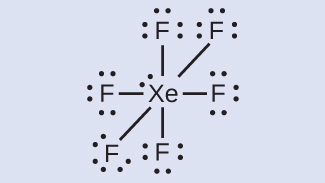# Question #e1d54

Apr 9, 2017Number of lone pair of electrons in $X e {F}_{2}$ is 3Number of lone pair of electrons in $X e {F}_{4}$ is 2Number of lone pair of electrons in $X e {F}_{6}$ is 1